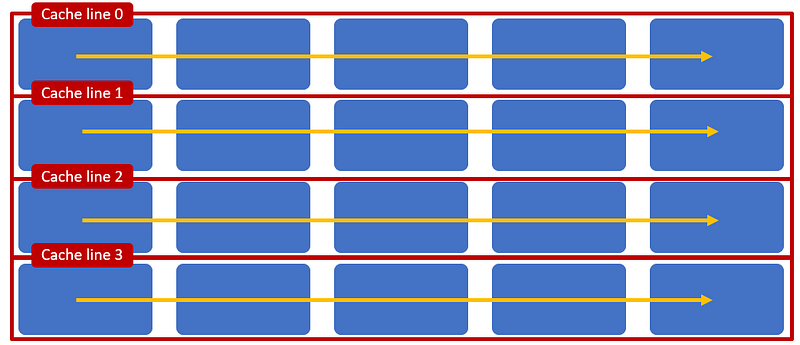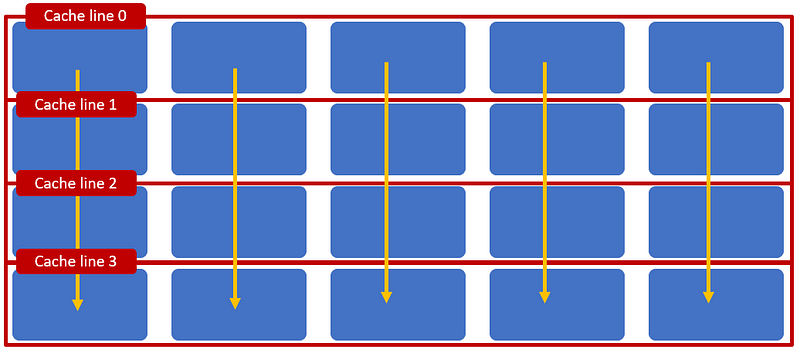# Optimizing Memory Access With CPU Cache

· Performance Zone · Tutorial
Save
12.33K Views

Nowadays, developers pay less attention to performance because hardware has become cheaper and stronger. If developers understand some basic knowledge about CPU and memory, they can avoid simple mistakes and it is easy to improve the performance of their code.

At the end of this article, I also ask a question that I do not know the answer to, so any suggestions are welcome!

## Cache Line

Before CPUs can run instructions or access data, it has to be loaded from memory to the CPU's cache (L1-L2-L3). Those caches don't read/write every single byte but by sequential bytes, which are called the cache line. The size of the cache line is a variable by each kind of the CPU and its technologies; most of the cache line has a size of 64 bytes. This mechanism increases copy speed between memory and CPU's cache but can cause some serious issues to performance.How cache lines work (credit)

## False Sharing

If two or more CPUs (or threads) read and write to two values in the same cache line, the cache line will be "dirty" and all CPUs have to reload the entire cache line to their caches. This is called false sharing, as shown in the above image. False sharing forces the CPUs to perform redudant loading data from memory and then decreases performance. This issue becomes more serious when more threads involve. We can solve this issue by telling the compiler how to store values. With C#, we can declare:

``````[StructureLayout(LayoutKind.Explicit)]
struct Data
{
[FieldOffset(0)]
public int x;

[FieldOffset(64)]
public int y;
}``````

Java 8 supports the annotation `@Contended` to do the similar job.

``````public class PaddedObject{
@Contended
public volatile long valueA;
public volatile long valueB;
}``````

With other versions of Java, we can put some redundant variables to achive the same output.

``````public class PaddedObject{
public volatile long v1,v2,v3,v4,v5,v6,v7;
public volatile long value;
public String toString() { // don't allow JVM remove unuse variables
return value + v1 + v2 + v3 + v4 + v5 + v6 + v7 + "";
}
}``````

Benchmarking result

 Benchmark Mode Cnt Score Error Units TestFalseSharing.padding avgt 200 5.933 ± 0.007 ms/op TestFalseSharing.unpadding avgt 200 27.177 ± 0.659 ms/op

## Multidimensional Arrays Access

The compiler can store arrays in memory as row-major order and column-major order; we can gain a lot from performance if we understand how arrays are stored.``````int[][] array = new int[size][size];
for(int i = 0; i < array.length; i++)
for(int j = 0; j < array.length; j++)
array[i][j] = i + j;``````

Row-major travel (credit)``````int[][] array = new int[size][size];
for(int i = 0; i < array.length; i++)
for(int j = 0; j < array.length; j++)
array[j][i] = i + j;``````

Column-major travel (credit)

We can see that, if arrays are stored as row-major order and we also travel in the same way, we can take advantage of the cache line mechanism. But in some cases, we have no choice other than to travel with a column-major order. Then, we can use loop blocking (or loop tiling).Loop blocking access (credit)

``````int result = 0;
int blockSize = 32;
for(int ii = 0; ii < 1024; ii+=blockSize)
for(int jj = 0; jj < 1024; jj+=blockSize) {
int i_upper = (ii + blockSize ) < 1024 ? (ii + blockSize ): 1024;
int j_upper = (jj + blockSize ) < 1024 ? (jj + blockSize ): 1024;
for(int i = ii; i < i_upper; i++)
for(int j = jj; j < j_upper; j++) {
result = state.data1[j][i];
}
}``````

## Array of Object

One time, I curiously compared the performance between an array of `int` and an array of `Integer`. The benchmark script is simple:

``````@Benchmark
@BenchmarkMode(Mode.AverageTime)
@OutputTimeUnit(TimeUnit.NANOSECONDS)
public int intArray(BenchMarkState state){
int result = 0;
for(int i = 0; i < state.size; i++) {
result = state.data1[i];
}
return result;
}
@Benchmark
@BenchmarkMode(Mode.AverageTime)
@OutputTimeUnit(TimeUnit.NANOSECONDS)
public int integerArray(BenchMarkState state){
int result = 0;
for(int i = 0; i < state.size; i++) {
result = state.data2[i].intValue();
}
return result;
}``````

We know that `Integer[]` is an array of pointers and travel on `Integer[]` is as fast as`int[]`. But if we need to access data of each Integer object, we need to access to allocated memory of the `Integer` object, and the JVM cannot guarantee where it is. The benchmark result shows that access to the value of the `Integer` object from an array is much slower than from an array of `int`.

``````//   size = 10240000
//Benchmark                         Mode  Cnt        Score       Error  Units
//TestObjectArray.intArray      avgt  200   213709.132 ±  4884.065  ns/op
//TestObjectArray.integerArray  avgt  200  2521451.187 ± 14602.431  ns/op``````

But if we reduce the size of the array to a small enough size (size = 16), we can see very different behavior. `Integer[]` is faster than `int[]`. IMHO, arrays with a small size enough can fit into the CPU L2 cache, but I cannot find an answer for this result. Do you have any ideas? If so, please let me know in the comments below.

``````//size = 16
//Benchmark                         Mode  Cnt  Score   Error  Units
//TestObjectArray.intArray      avgt  200  8.852 ± 0.256  ns/op
//TestObjectArray.integerArray  avgt  200  5.774 ± 0.019  ns/op``````

## Conclusion

With basic knowledge about CPU and memory, we can avoid some bad performance code. There is more Java benchmark code at my GitHub repo. Be sure to check it out!

Topics:
memory access pattern, memory allocation, memory cache, performance, array, integer, int, object, c#

Opinions expressed by DZone contributors are their own.